1. 在合适的坐标系下老鼠移动轨迹的方程；
2. 老鼠移动的路程。

#### 解法一

$A(r,\theta), B (r,\theta+\frac{\pi}{4})$

$(rcos \theta,rsin \theta)$

$(-rsin\theta,rcos\theta)$

\begin{align} m& =\frac{rcos\theta-rsin\theta}{-rsin\theta-rcos\theta}\\ & =\frac{sin\theta-cos\theta}{sin\theta+cos\theta}\\ & =\frac{tan\theta-1}{tan\theta+1} \end{align}

\begin{align} m & = \frac{dy}{dx} \\ & =\frac{\frac{dr}{d\theta}sin\theta+rcos\theta}{\frac{dr}{d\theta}cos\theta-sin\theta}\\ & = \frac{\frac{dr}{d\theta}tan\theta+r}{\frac{dr}{d\theta}-rtan\theta} \end{align}

$\frac{tan\theta-1}{tan\theta+1}=\frac{r'tan\theta+r}{r'-rtan\theta}$

$r'(1+tan^2\theta)=-r(1+tan^2\theta)$

$r'=-r$

$r(\theta)=Ce^{-\theta}$

$r(\frac{\pi}{4})=\frac{1}{2}\sqrt{2}a$

$C=\frac{\sqrt{2}}{2}ae^{\pi/4}$

$r(\theta)=\frac{a\sqrt{2}}{2}e^{\pi/4-\theta}$

$r(\theta)=\frac{a\sqrt{2}}{2}e^{\alpha-\theta},\\ \alpha=\frac{\pi}{4},\frac{3\pi}{4},\frac{5\pi}{4},\frac{7\pi}{4}$

\begin{align} L & = \int_{\pi/4}^{ + \infty } {\sqrt{r^2+(\frac{dr}{d\theta})^2}d\theta} \\ & = \int_{\pi/4}^{ + \infty } {\sqrt{a^2e^{\pi/2-2\theta}}d\theta} \\ & = \int_{\pi/4}^{ + \infty } {ae^{\pi/4-\theta}d\theta} \\ & = -a \cdot e^{\pi/4-\theta}|_{\pi/4}^{+\infty}\\ & = -a(0-1)\\ & = a \end{align}

$(\frac{dr}{d\theta})^2=(-r)^2=r^2=\frac{a^2}{2}e^{\pi/2-2\theta}$

#### （并不十分严谨的）解法二####$n=100$ 时的过程。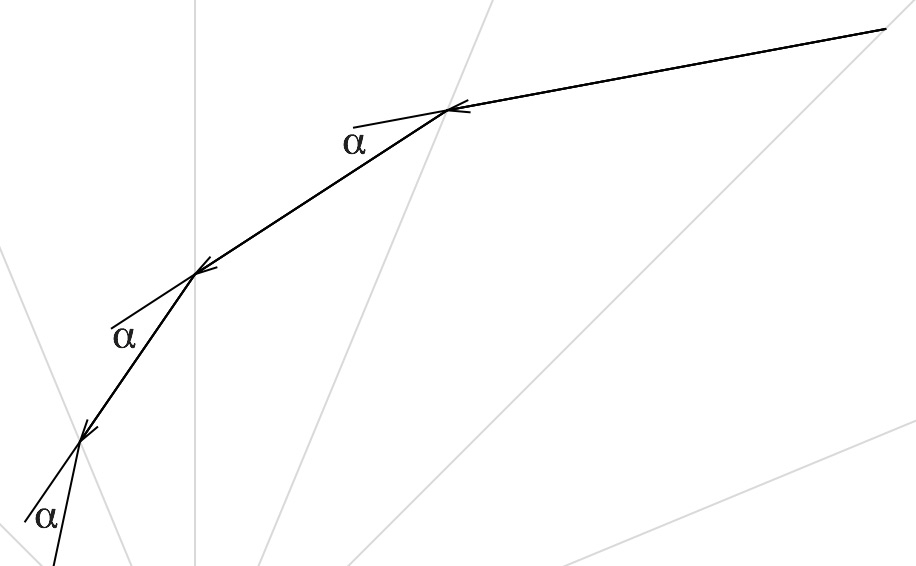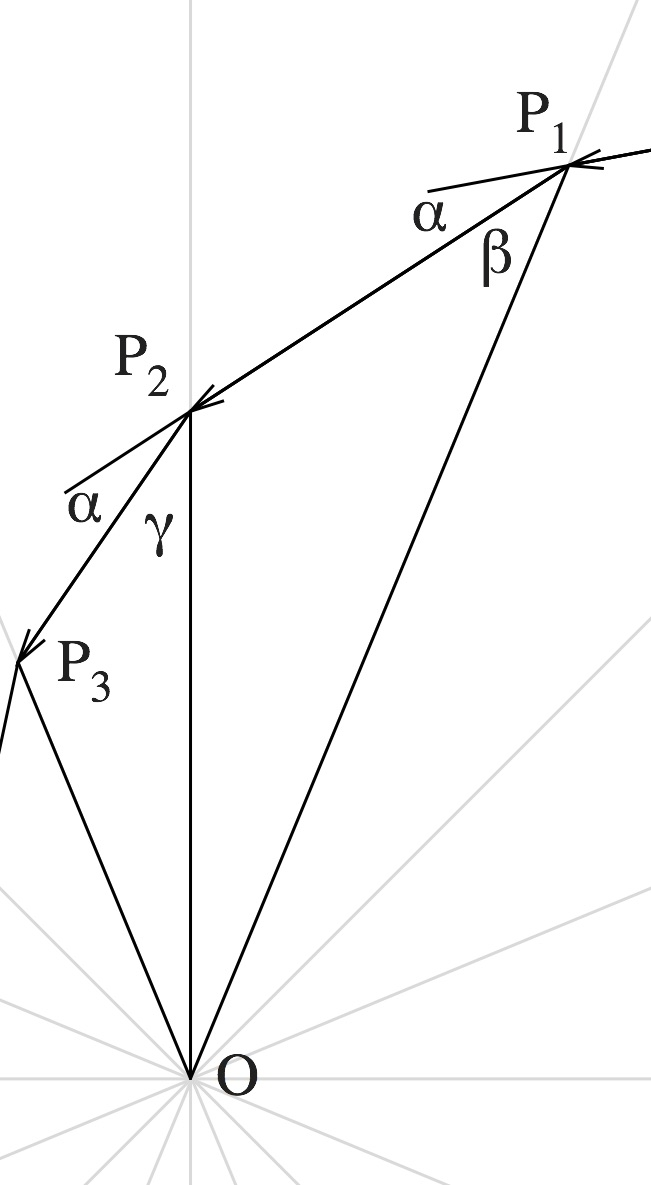$\alpha+\gamma=\alpha+\beta$

$r=\frac{a\sqrt{2}}{2}e^{\pi/4-\theta}$

#### 结论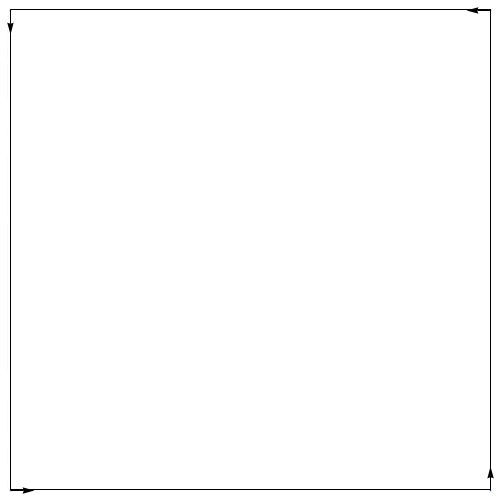#### 然后呢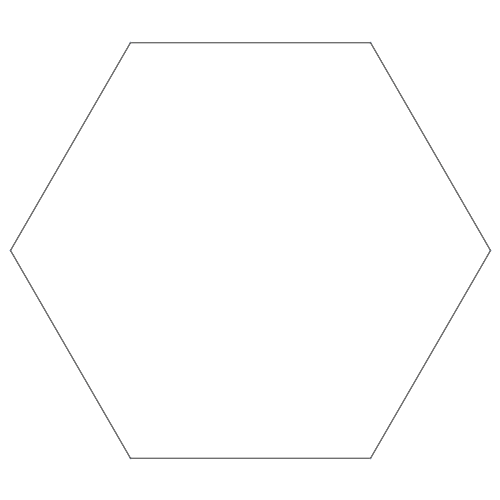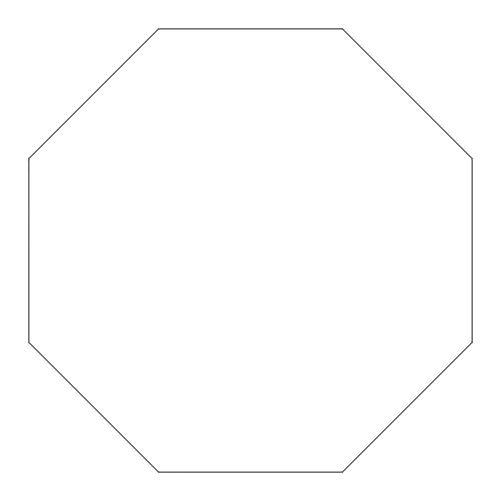#### 思考####

• 结论然后呢中动图的表现方式有什么不同？结合下面的配图，你能联想到什么？
• 为什么任意中心位于原点的对数螺线的方程都能用$r=ae^{b\theta}$来表示？能不能在别的位置添加新的参数？
• 当四只老鼠的速度不相同时，轨迹形状会发生怎样的变化？尝试画出大致图形。
• 解法二解法一相比，无需使用较为高深的微积分，但同时也没有给出第二小问的解。你能不用微积分解出第二小问吗？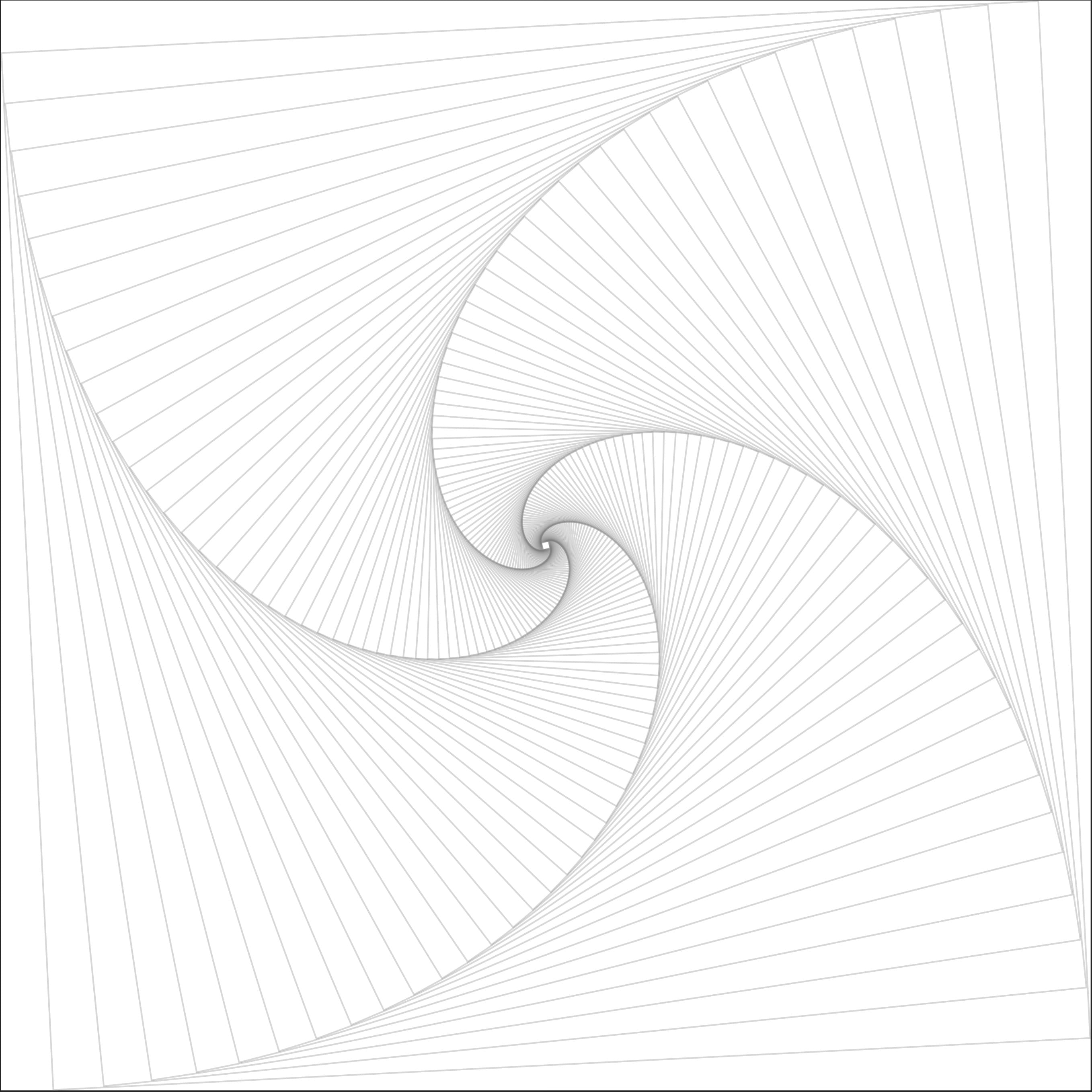#### 其它

• 这个问题是我在某天蹭选修课《数学探究与欣赏》的时候听到的。起初我以为轨迹会是贝塞尔曲线的一部分（因为其定义与题目条件形式有些相似，但不一样）。
• 对数螺线又叫等角螺线。
• 这个问题是被称作追逐曲线的一类问题中的一个。
• 插图使用 Mathematica 与 Sketch 绘制，版权所有。